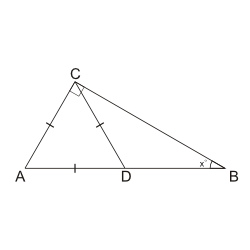We cover every section of the GMAT with in-depth lessons, 5000+ practice questions and realistic practice tests.

## Up to 90+ points GMAT score improvement guarantee

### The best guarantee you’ll find

Our Premium and Ultimate plans guarantee up to 90+ points score increase or your money back.

## Master each section of the test

### Comprehensive GMAT prep

We cover every section of the GMAT with in-depth lessons, 5000+ practice questions and realistic practice tests.

## Schedule-free studying

### Learn on the go

Study whenever and wherever you want with our iOS and Android mobile apps.

# Triangles: The Angles in a Triangle Sum Up to 180°If triangle $$ACD$$ is equilateral and triangle $$ACB$$ is right as shown above, what is the value of $$x^\circ$$?
Correct. [[snippet]] Given that $$AC = AD = CD$$, triangle $$ACD$$ is an equilateral triangle with all three angles equal to $$\frac{180^\circ}{3} = 60^\circ$$. So >$$x^\circ = 180^\circ - \angle ACB - \angle DAC = 180^\circ - 90^\circ - 60^\circ = 30^\circ$$.
Incorrect. [[snippet]] You might have gotten this answer if you found $$\angle CDB$$ instead of $$\angle CBD$$.
Incorrect. [[snippet]] Carefully check your calculations.
Incorrect. [[snippet]] This is the measures of the angles in triangle $$ACD$$, not the value of $$x$$.
Incorrect. [[snippet]] Carefully check your work.
30°
45°
60°
90°
120°